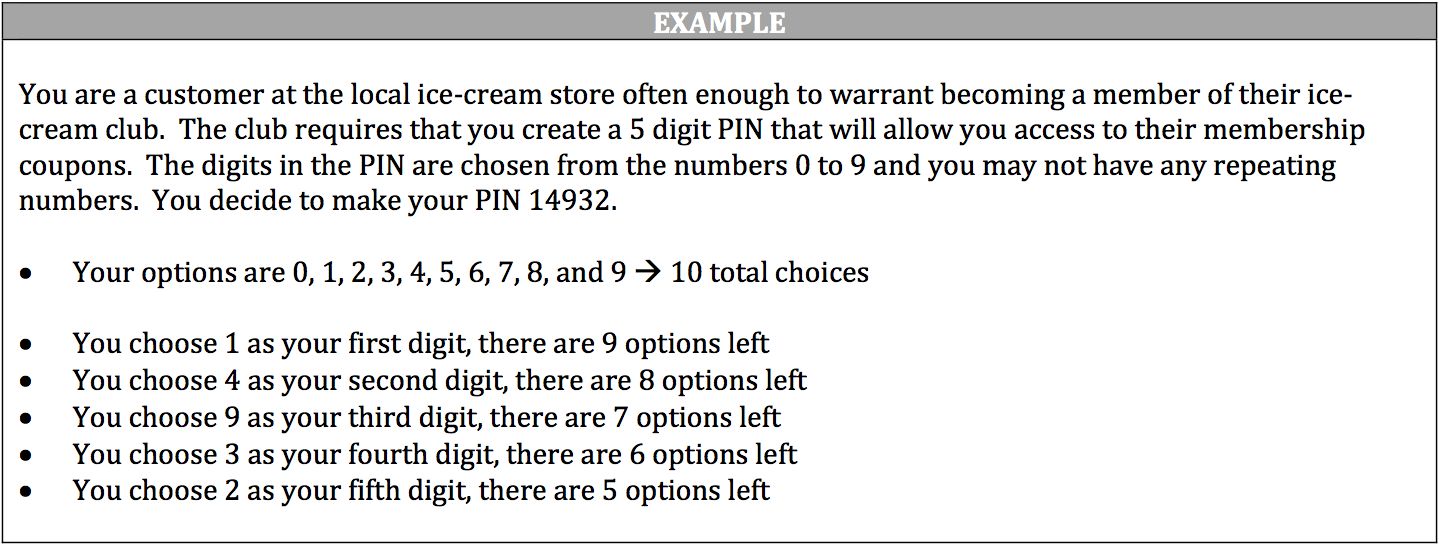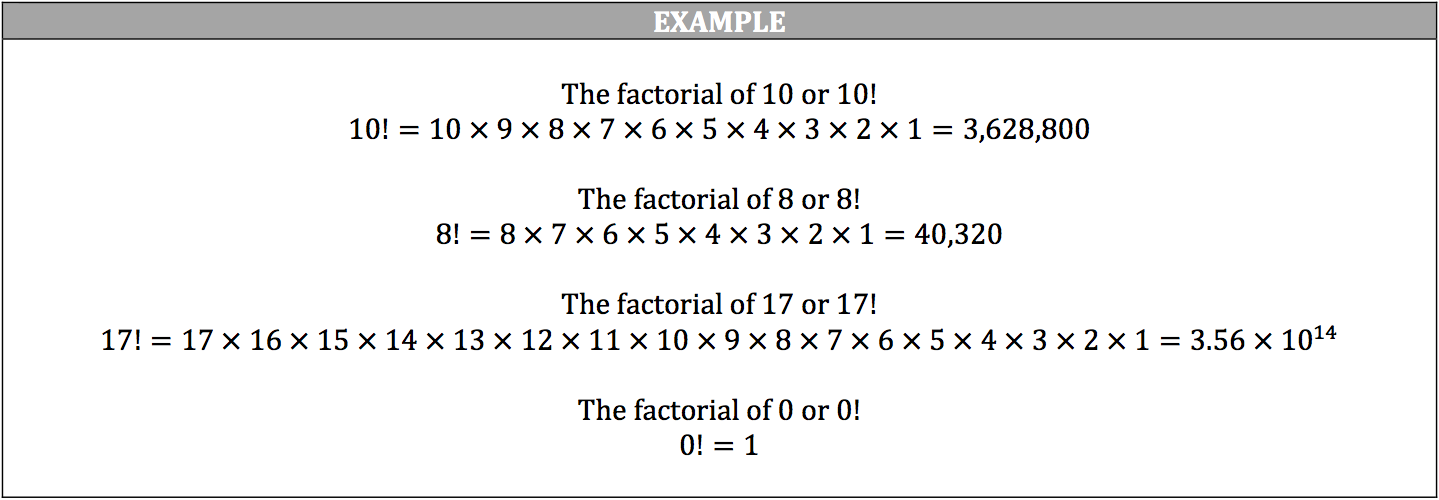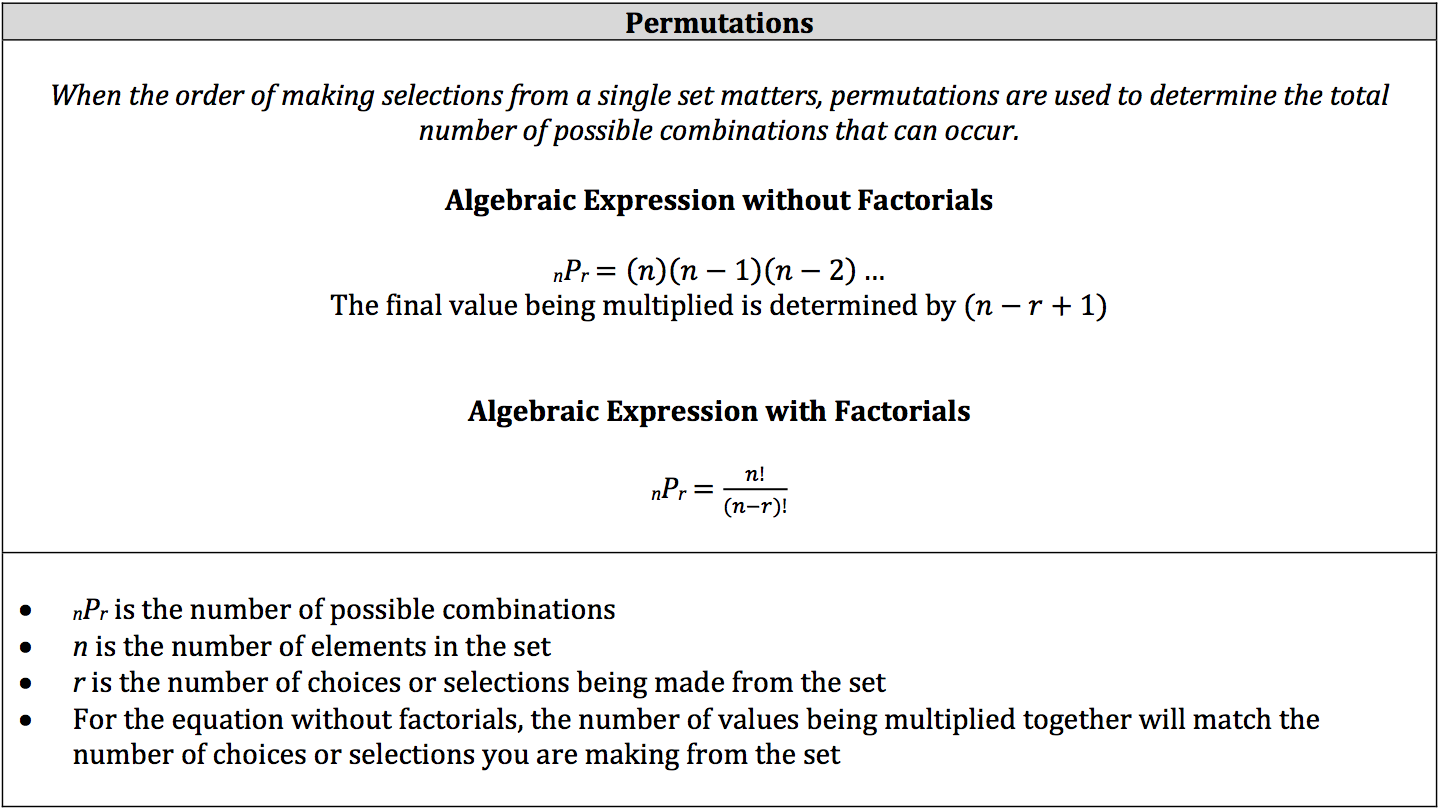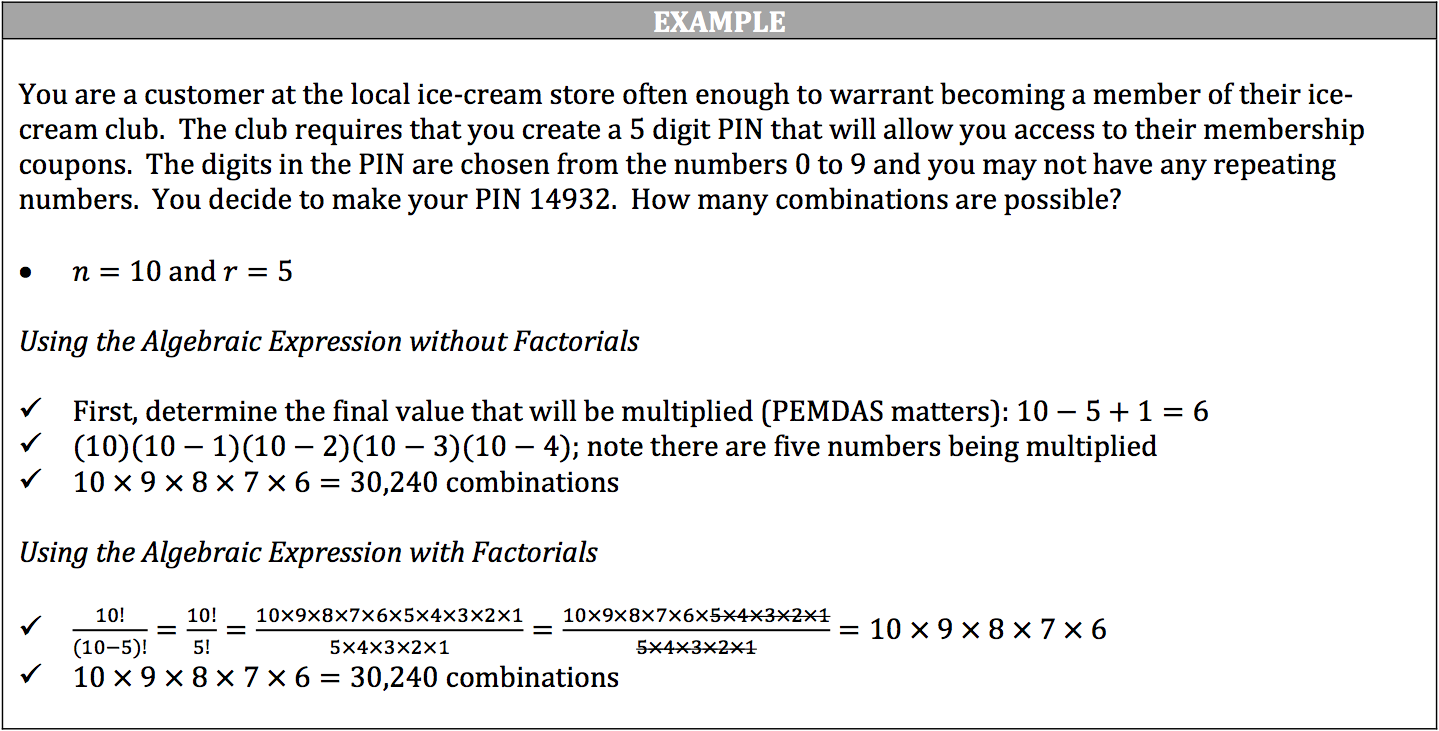Permutations are a variation of the fundamental counting principle except that you are determining a certain number of combinations from a single set. More importantly, the order in which you choose elements from the set matters. For example, you would use permutations when making a password (123 is not the same as 321). Also, when you select an element from a set, you reduce the number of elements by 1 each time.You can solve this question using an algebraic expression with or without factorials. A factorial is represented by the exclamation mark (!) and means the number will be multiplied by all integers between itself and 1 (inclusive). In other words, if you have 5!, then the number 5 will be multiplied by 4, 3, 2, and 1. It is easiest to multiply the values in descending order, but you can also start with 1 and multiply up to 5. Do not include zero as it would make the whole thing equal zero.

Oddly enough, however, the factorial of zero or 0! is equal to 1. The reason for this is that zero represents an empty set, which is always included in the possible combinations. Thus, if the set is empty, there is only one way the event will happen. The formula for subsets (S = 2) also proves this to be true: n = 0; 2⁰ = 1.